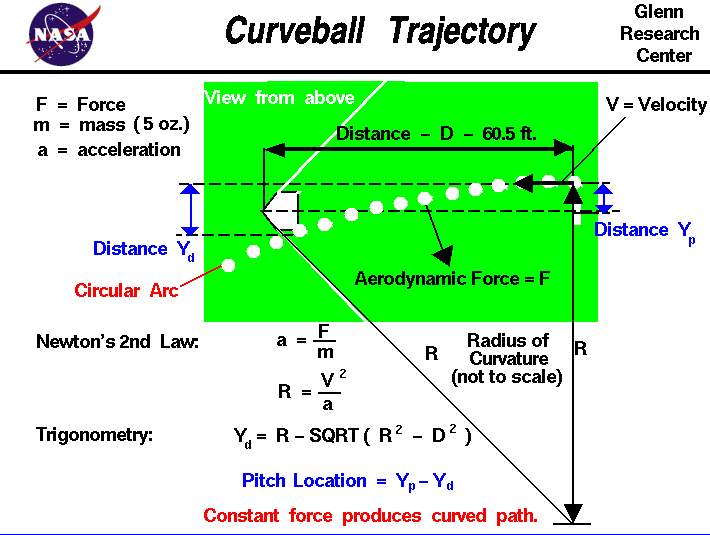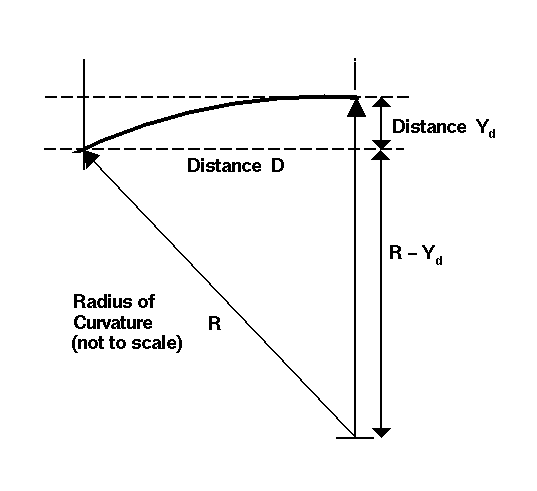+ Text Only Site
+ Non-Flash Version
+ Contact GlennAll that is necessary to create lift is to turn a flow of air. The airfoil of a wing turns a flow, but so does a spinning baseball. The details of how the force is generated are fairly complex, but the magnitude of the force F depends on the radius of the ball b, the spin of the ball s, the velocity V of the pitch, the density r of the air, and an experimentally determined lift coefficient Cl. F = Cl * 4 /3 * (4 * pi^2 * r * s * V * b^3) where pi is the number 3.14159 .. the ratio of the circumference to the diameter of a circle. As the force acts on the ball, it is deflected along its flight path. If we neglect the viscous forces on the ball, which slow it down and change the magnitude and direction of the force, we have a constant force always acting perpendicular (at a right angle) to the flow direction. The resulting flight path is a circular arc. On the figure, we see the trajectory of the baseball as it moves from the pitching mound to the plate from right to left. Each white dot is the location of the ball at .05 second intervals. It gets to the plate pretty fast! The radius of curvature R of the flight path depends on the velocity V of the pitch and the acceleration a produced by the side force. R = V^2 / a We can determine this acceleration from Newton's second law of motion using the force for a spinning ball and the mass (m) of the baseball (5 oz.). a = F / m Since the radius of curvature depends on the force, all the factors that affect the force will also affect the trajectory. Collecting all the information into one equation: R = (3 * m * V) / (16 * Cl * r * s * b^3 * pi^2) We can use this equation to make some predictions about the trajectory of a spinning ball. Higher spin s produces a smaller radius of curvature R and a sharper curve. Higher velocity V produces a larger radius of curvarture and a straighter curve. A ball with a smaller mass, like a ping-pong ball, has a lower radius of curvature and curves more. At higher altitudes, the density r is lower producing a larger radius of curvature and a straighter path. It's very hard to make a ball curve on Mt. Everest, no matter how much it spins. That is good for golfers and bad for baseball pitchers. The altitude effect helps to explain the high batting averages and poor earned run averages for the Colorado Rockies who play 81 games a year at a high altitude ball park. It is much harder to throw a curve ball at Coors Field than at Dodger Stadium. Knowing the radius of curvature and the distance from the pitching mound to home plate, we can also calculate the distance that the ball is deflected (Yd) along the flight path.Here we have a sketch of the geometry of the pitch. There is a right triangle formed by the radius of curvature R, the distance to the plate D at the top, and the radius of curvature minus the deflection distance R - Yd on the right. We can then use the Pythagorean Theorem to relate the sides of this triangle: R^2 = D^2 + (R - Yd)^2 Now let's do a little algebra: R^2 - D^2 = (R -Yd)^2 sqrt(R^2 - D^2) = R -Yd Yd = R - sqrt(R^2 - D^2) The pitcher can release the ball at different distances from the center of the plate (Yp). The difference between Yp and Yd will give the final location of the pitch relative to the center of the plate. You can investigate the effect of aerodynamics on throwing a curve ball by using the CurveBall Java Applet. Have fun ! You can use the browser "Back" button to return to this page. If you want your own copy of CurveBall to play with, you can download it for free. Activities:Guided ToursCurveBall - Baseball Simulation:Navigation ..NASA Baseball Home Page Beginner's Guide Home Page+ Inspector General Hotline + Equal Employment Opportunity Data Posted Pursuant to the No Fear Act + Budgets, Strategic Plans and Accountability Reports + Freedom of Information Act + The President's Management Agenda + NASA Privacy Statement, Disclaimer, and Accessibility CertificationEditor: Nancy Hall NASA Official: Nancy Hall Last Updated: May 05 2015 + Contact Glenn